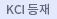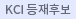#검색
다국어 입력

http://chineseinput.net/에서 pinyin(병음)방식으로 중국어를 변환할 수 있습니다.

변환된 중국어를 복사하여 사용하시면 됩니다.

예시)
• 中文 을 입력하시려면 zhongwen을 입력하시고 space를누르시면됩니다.
• 北京 을 입력하시려면 beijing을 입력하시고 space를 누르시면 됩니다.
닫기

검색결과 좁혀 보기

• 좁혀본 항목 보기순서

• 원문유무
• 음성지원유무
• 원문제공처
• 등재정보
• 학술지명
• 주제분류
• 발행연도
• 작성언어
• 저자

오늘 본 자료

• 오늘 본 자료가 없습니다.
더보기
• 무료
• 기관 내 무료
• 유료
•THE SIMPLE FORMULA OF CONDITIONAL EXPECTATION ON ANALOGUE OF WIENER MEASURE

In this note, we establish the uniqueness theorem of conditional expectation on analogue of Wiener measure space for given distributions and prove the simple formula of conditional expectation on analogue of Wiener measure which is essentially similar to Park and Skoug's formula on the concrete Wiener measure.

•FGK INEQUALITY ON THE ANALOGUE OF WIENER SPACE

This work aims at proving the FKG inequalities for the analogue of Wiener functionals with special orders on the analogue of Wiener space.

•THE GENERALIZED ANALOGUE OF WIENER MEASURE SPACE AND ITS PROPERTIES

In this note, we introduce the de¯nition of the gener-alized analogue of Wiener measure on the space C[a, b] of all real-valued continuous functions on the closed interval [a, b], give several examples of it and investigate some important properties of it - the Fernique theorem and the existence theorem of scale-invariant mea-surable subsets on C[a, b].

•The arcsine law in the generalized analogue of wiener space

In this note, we prove the theorems in the generalized analogue of Wiener space corresponding to the second and the third arcsine laws in either concrete or analogue of Wiener space [1,2,7] and we show that our results are exactly same to either the concrete or the analogue of Wiener case when the initial condition gives either the Dirac measure at the origin or the probability Borel measure.

•The Rotation Theorem on Analogue of Wiener Space

Bearman's rotation theorem is not only very importantin pure mathematics but also plays the key role for various researchareas, related to Wiener measure. In 2002, the author and professorIm introduced the concept of analogue of Wiener measure, a kindof generalization of Wiener measure and they presented the severalpapers associated with it. In this article, we prove a formula onanalogue of Wiener measure, similar to the formula in Bearman'srotation theorem.

•PROBABILITIES OF ANALOGUE OF WIENER PATHS CROSSING CONTINUOUSLY DIFFERENTIABLE CURVES

Let φ be a complete probability measure on R, let mφbe the analogue of Wiener measure over paths on [0, T] and let f(t)be continuously differentiable on [0, T]. In this note, we give theanalogue of Wiener measure mφ of {x in C[0, T]|x(0) < f(0) andx(so) ≥ f(so) for some s0 in [0, T]} by use of integral equationtechniques. This result is a generalization of Park and Paranjape's1974 result.

•The Dobrakov integral over paths

In 2002, the author introduced the definition and its properties of an analogue of Wiener measure over paths. In this article, using these concepts, we will derive an operator-valued measure over paths and will investigate the properties for integral with respect to the measure. Specially, we will prove the Wiener integral formula for our integral and give some example of it. In 2002, the author introduced the definition and its properties of an analogue of Wiener measure over paths. In this article, using these concepts, we will derive an operator-valued measure over paths and will investigate the properties for integral with respect to the measure. Specially, we will prove the Wiener integral formula for our integral and give some example of it.

•The measure-valued Dyson series and its stability theorem

In this article, we establish the existence theorem for measure-valued Dysonseries and show that it satisfies the Volterra-type integral equation. Furthermore,we prove the stability theorems for measure-valued Dyson series.

•ANALOGUE OF WIENER INTEGRAL IN THE SPACE OF SEQUENCES OF REAL NUMBERS

Let $T > 0$ be given. Let $(C[0,T], m_\varphi )$ be the analogue of Wiener measure space, associated with the Borel probability measure $\varphi$ on $\mathbb R$, let $(L_2 [0,T], \widetilde{\omega})$ be the centered Gaussian measure space with the correlation operator $(- \frac{d^2}{dx^2})^{-1}$ and $(\ell_2 , \widetilde{m})$ be the abstract Wiener measure space. Let $\mathcal U$ be the space of all sequence $\langle c_n \rangle$ in $\ell_2$ such that the limit $\lim_{m\to \infty} \frac{1}{m+1} \sum_{n=0}^m \sum_{k=0}^n c_k \cos \frac{k \pi t}{T}$ converges uniformly on $[0,T]$ and give a set function $m$ such that for any Borel subset $G$ of $\ell_2$, $m(\mathcal U \cap P_0^{-1} \circ P_0 (G))=\widetilde{m}(P_0^{-1} \circ P_0 (G))$. The goal of this note is to study the relationship among the measures $m_\varphi , \widetilde{\omega}, \widetilde{m}$ and $m$.

•